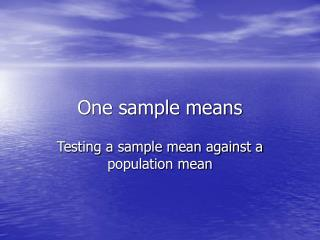Download PresentationOne sample meansOne sample means - PowerPoint PPT Presentation

Download PresentationOne sample means
An Image/Link below is provided (as is) to download presentation

Download Policy: Content on the Website is provided to you AS IS for your information and personal use and may not be sold / licensed / shared on other websites without getting consent from its author. While downloading, if for some reason you are not able to download a presentation, the publisher may have deleted the file from their server.

- - - - - - - - - - - - - - - - - - - - - - - - - - - E N D - - - - - - - - - - - - - - - - - - - - - - - - - - -
Presentation Transcript

1. One sample means Testing a sample mean against a population mean

2. Hypothesis testing for one population means • We have measured a sample, and have a mean and SD, interval or ratio level data • Does the sample differ significantly from the population? • Review of statistical significance: could this difference be chance, or is there a real difference?

3. Statistical significance • Usual rule of thumb: there must be less than a 5% probability (1 in 20) that this result could have been obtained by chance • Null hypothesis: the mean of the sample is not different from that of the population • Non-directional alternative hypothesis: there is a difference—which one is higher is not known

4. Statistical significance • Directional hypothesis: there is a difference and we specify whether the sample or the population will have the higher mean.

5. Testing a sample • Assuming the sampling means form a normal distribution (central limit theorem) we can use z scores to determine if a sample differs significantly from a population

6. Formula: Calculate Z statistic • Z = (Mean of the sample-mean of population)/SD/square root of n (number of subjects) • We can then use this z score to determine the likelihood of getting a result significantly different from the population • (the Z statistic should not be confused with z scores)

7. Example • The mean IQ of our training school population is 87 with a sample size (n) of 256 • The mean in the general population is 100 with a SD of 15 • Z = 87-100/(15/sqrt of n) • -13/(15/16) = 13/.9375 = 13.8 • Statistically significant

8. Example • See table on p. 284 for determining significance • Suppose that the mean is 102, sample size 100 • Z = 102-100/(15)/10) = 1.33 • Not statistically significant, the sample if from the same population